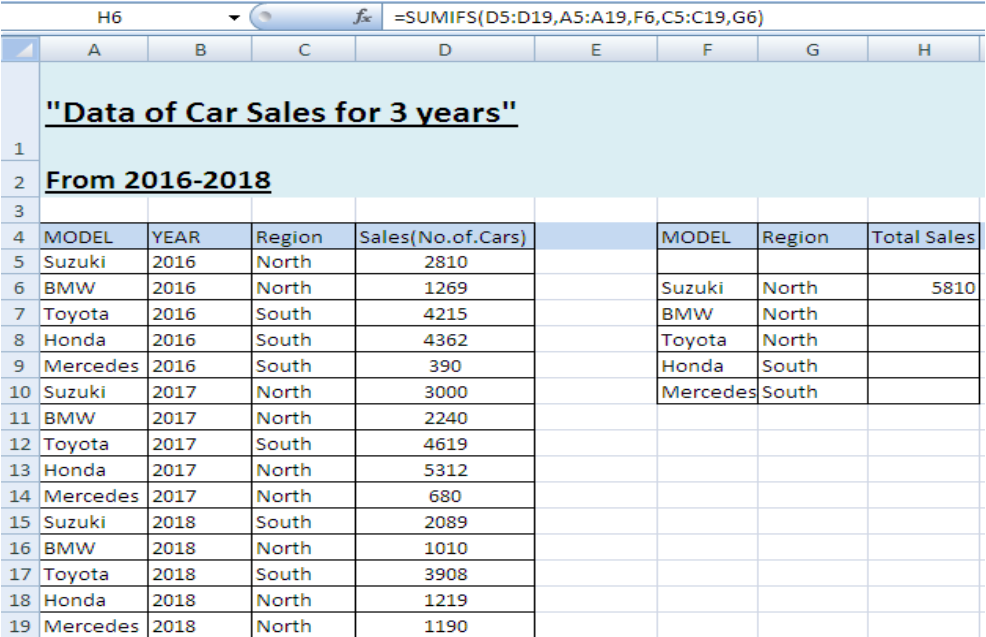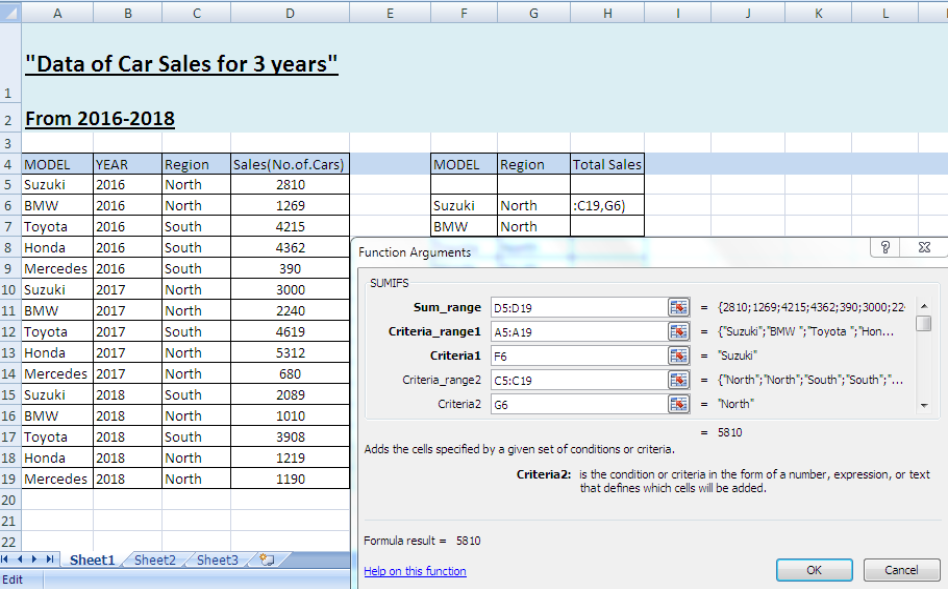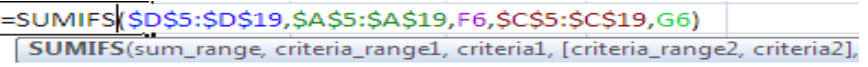Get instant live expert help with Excel or Google Sheets“My Excelchat expert helped me in less than 20 minutes, saving me what would have been 5 hours of work!”

#### Post your problem and you’ll get expert help in seconds.

Your message must be at least 40 characters
Our professional experts are available now. Your privacy is guaranteed.

# Working with the SUMIF Function and Two Conditions

SUMIF: SUMIF is a function in Excel used to add all the numbers in a range based on one criteria/condition. In case of two or more criteria’s/conditions, the formula used is:

Formula: `=SUMIFS(sum_range, criteria_range1, criteria1, [criteria_range2, criteria2])`

SUMIF Function in Excel can be used in two ways:

• By putting SUMIF Formula in the worksheet cell and entering values in it.
• By using the option of before the formula bar.

Let’s see how we can calculate the sum of a range with two conditions by using two different methods:

## Method 1Figure 1

According to the given figure, we’re going to calculate total sales of cars for 3 years based on the model of the car and the region where the cars are sold.

• Select cell H6, use SUMIF formula and insert the values in the formula.

Formula: `=SUMIFS(sum_range,criteria_range1,criteria1,criteria_range2,criteria2)`

(Cell D5:D19 is referred as sum_range, A5:A19 is criteria_range1, F6 is criteria1, C5:C19 is criteria_range2, and G6 is criteria2.)

• Press Enter after entering the values and we’ll get the total sales of Suzuki in the North region.
• By clicking on H6, we can see the SUMIF formula in the formula bar.
• Now lock the formula by pressing F4, but remember not to lock “criteria1” and “criteria2” as they are different for every cell of total sales.
• Click H6 and drag it to H10 to get total sales of all the cars for the mentioned regions.

## Method 2Figure 2

The steps to get the same result with the same formula are as follows:

• Write =SUMIFS in H6, then click Fx  in the toolbar before the formula bar.
• A small window will open in the excel worksheet carrying all the titles of the formula.
• Fill the titles one by one and press OK.
• We’ll get the same values obtained by using method 1.
• Lock the formula and drag it to H10, all the values of total sales will be calculated automatically meeting both the conditions.

## Notes

• While locking the formula, Select the formula except criteria1 “F6” and criteria2 “G6”
• Otherwise, results won’t be the same.Figure 3

You can see this is a locked formula in case of the given example.

Most of the time, the problem you will need to solve will be more complex than a simple application of a formula or function. If you want to save hours of research and frustration, try our live Excelchat service! Our Excel Experts are available 24/7 to answer any Excel question you may have. We guarantee a connection within 30 seconds and a customized solution within 20 minutes.

Solution examplesamending a formula so that there is another criteria and if it does not meet this criteria then we stick with the original criteria
Solved by A. B. in 40 minsI am looking for a formula to SUM column L, if Column A matched the relevant date and column C matches the name
Solved by Z. Y. in 16 minsI am trying to utilize a complicated sumif or sumifs formula that can pull a total amount from a date and a drop down box from another tab.
Solved by E. B. in 15 minsI have a spreadsheet that totals invoices. A bunch of cells at the bottom of the spreadsheet calculates totals based on which department the invoice is charged to. I'd like to add a function where it also considers the date (so if it's an invoice in January, it goes to a January total, February, etc.). But I can't figure out how to write it properly...
Solved by K. J. in 27 minsIf I want to write a formula that states" "If this name shows up K24, and this word shows up in J24 pull this number from M24" I can creat that formula - however I need to drag this down so it pulls from row 24 - 60 - is there way to do this?
Solved by F. D. in 40 mins